# Tokyo 2020 Betting II: Model Refinement and Feature Engineering

The second post in a series building towards betting on the Olympic track cycling. This time I’ll adapt the Bradley-Terry model to use a custom likelihood to better capture the competition format, and integrate new data fields to boost model performance.

Last time I introduced the basic model I’ll be using to predict the results of the track cycling Individual Sprint at Tokyo 2020.

This post will pick up where that ended, with the focus turning to building on the basic model: adapting it to handle more of the specifics of the sport.

The table below summarises the changes I’ll explore:

Post Model Description
Tokyo 2020 Betting I bt1 Basic Bradley-Terry
bt2 Informative (Gamma) Prior
Tokyo 2020 Betting II bt3 Match Likelihood
bt5 Time Dependent Strengths
bt6 Qualifying Times

I’ll present the model developments in the order I made them - which matches the above - rather than the level of impact they have on evaluation metrics. In the conclusion I’ll reflect on how I could have improved my approach to prioritising the developments.

### Assumptions

This post makes some assumptions about you!

I’ll assume you’ve read the previous post in the series (though this might hold-up as a stand-alone): I won’t recap the data or basic model.

The model variants will rely on a few technical tools that I’ll recap, but not fully introduce:

• the role of the likelihood in regression models,

• hierarchical (random) effects, and

• Gaussian processes.

If you’re interested in reading the underlying code, this is in R and Stan.

## The Match Likelihood

The Bradley-Terry model assumes matches are won or lost, with no delineation of the scale of a win.

In the early heats of the individual sprint this is an accurate assumption: the format is a knockout where a single sprint is contested.

From the quarterfinals, matches are contested in a best-of-three format which introduces a scale to winning: if $$p$$ is the probability that a given athlete wins a single sprint, the outcomes that they win a best-of-three match are:

Scenario Sprints Contested Prob. Occurrence
Wins first two sprints outright 2 $$p^2$$
Wins first and third, loses second 3 $$p(1-p)p$$
Loses first, wins second and third 3 $$(1-p)p^2$$
Total $$p^2 + 2p^2(1-p)$$
Show code
p_best_of_three <- ggplot(tibble(p=c(0,1)), aes(p)) +

stat_function(
aes(linetype = '2', color = '2'),
fun = function(p){ p^2  },
size=1, color = infreq_palette["darkblue"]
) +

stat_function(
aes(linetype = '3', color = '3'),
fun = function(p){(2*p^2*(1-p))},
size=1, color = infreq_palette["darkblue"]
) +

stat_function(
aes(linetype = 'Total', color = 'Total'),
fun = function(p) p^2 + 2*p^2*(1-p) ,
size = 2, color = infreq_palette["orange"]
)  +

geom_text(
data = tribble(~p, ~y, ~text, ~color,
0.9, 0.60,'2 sprints', 'Total',
0.9, 0.31, '3 sprints','3',
0.92, 1.07, 'Total', '2'),
aes(p,y,label=text),
color = c(infreq_palette["darkblue"], infreq_palette["darkblue"], infreq_palette["orange"]),
size = c(5,5,6)
) +

scale_y_continuous(limits=c(0,1.2), breaks = seq(0,1.2,by=0.2)) +
coord_cartesian(ylim = c(0,1.1)) +
labs(x="Prob. Wins Sprint", y="Prob. Wins Match") +
scale_linetype_manual(values = c("solid", "dotted", "solid")) +
scale_color_manual(values = c(infreq_palette["darkblue"],infreq_palette["darkblue"],infreq_palette["orange"])) +
theme(legend.position = "none")

p_best_of_three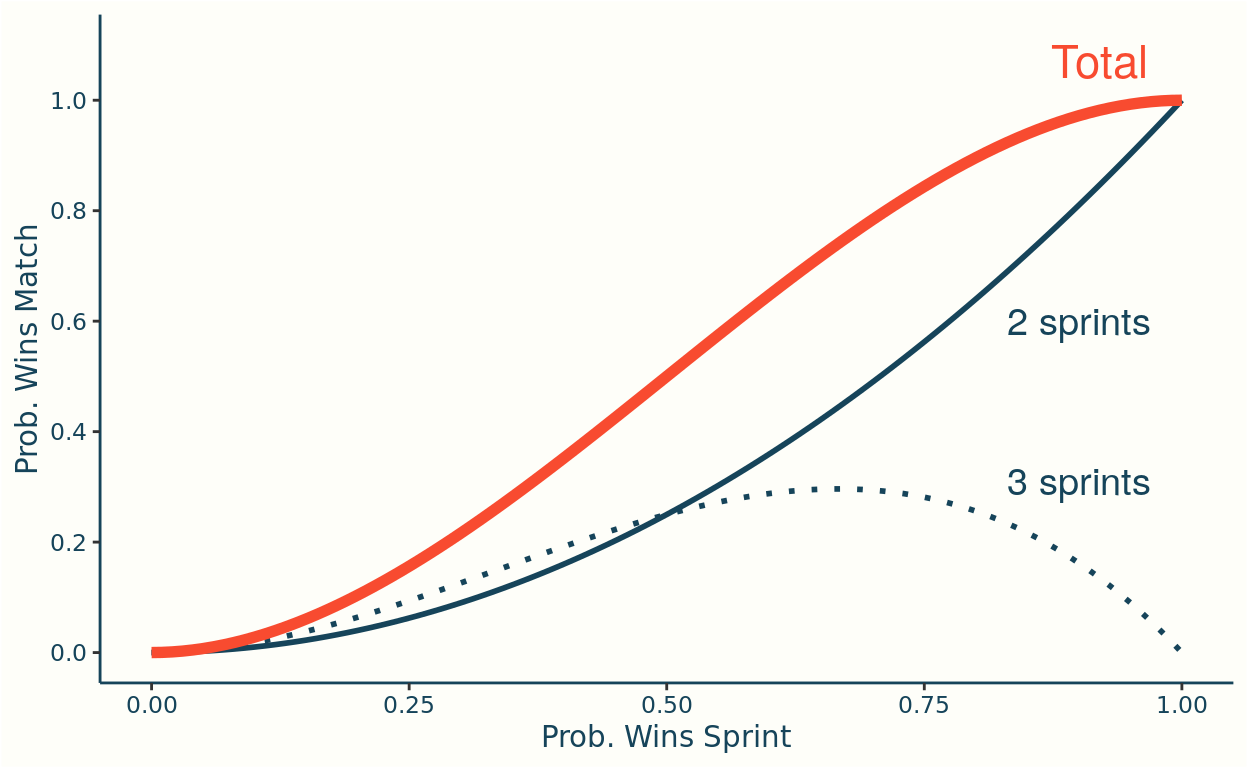The best-of-three format can be considered fairer than a single sprint, as it increases the probability that the stronger athlete wins.

I’ll adapt the Bradley-Terry model to use a custom likelihood: in early rounds this will be as before, but for later rounds it will take into account the best-of-three format.

One convenience I’ll introduce is to formulate the model from the perspective of the winning rider. In words the likelihood I’ll consider is:

Given that the winning athlete, $$w$$, beat the loser, $$l$$, in $$S$$ sprints: how likely is it that the strength difference between them is $$\delta = \alpha_w - \alpha_l$$?

To simplify the formula for the likelihood I’ll let $$p_\delta = \text{logit}^{-1}(\delta)$$ which, as covered in the last post, is the probability of winning a single sprint given the strength difference $$\delta$$.

The match likelihood is then defined as:

$L_{\text{match}}\big(\alpha_w - \alpha_l = \delta \, |\, S = s\big) = \begin{cases} p_\delta, & s = 1 \\ \\ p_\delta^2, & s = 2\\ \\ 2p_\delta^2 (1-p), & s = 3 \end{cases}$

The formula above is not a true likelihood per se, I’m using two convenient properties of the race format and the data, that allow this reduced definition:

Firstly, the case $$s=1$$ gets invoked on a separate subset of the data to the cases $$s=2,\,3$$. So really this is a separate likelihood (equivalent to the Bernoulli, logistic regression, model). Combining the two scenarios in one formula is a convenience as I can fit all the data to one model, rather than splitting out the cases.

Secondly, both likelihoods ($$s=1$$ case, and $$s=2,3$$) are presented from the perspective of the winning rider: i.e. we don’t have the cases $$s=-1,-2,-3$$. In reality these cases do exist - but by feeding the model data from the winning perspective the other scenarios won’t be induced.

BT3 \begin{align*} \bf{\text{Priors}}\\ \sigma & \sim \text{Gamma}(80,60) \\ \alpha_r & \sim \text{Normal}(0,\sigma) \\ \\ \bf{\text{Likelihood}}\\ \alpha_w - \alpha_l | S &\sim L_{\text{match}}(\alpha_r - \alpha_s | S) \end{align*}

Example Data
Show code
# read match data from remote _target store

matches_sample <-  matches %>%
filter(event == '2020 UCI TRACK WORLD CYCLING CHAMPIONSHIPS', round == 'Finals', gender == 'Women') %>%
mutate(across(everything(), as.character)) %>%
select(event, date, gender, round, rider_1,rider_2,rider_id_1,rider_id_2, winner_id,loser_id, sprints) %>%
slice(1)

matches_sample <- tibble(Field = names(matches_sample), Example = unlist(matches_sample[1,]))
matches_sample %>%
kable("pipe") %>%
kable_styling(full_width=FALSE, bootstrap_options = c("striped", "hover", "condensed"),font_size = 10)

Field Example
event 2020 UCI TRACK WORLD CYCLING CHAMPIONSHIPS
date 2020-02-28
gender Women
round Finals
rider_1 HINZE EMMA
rider_2 VOINOVA ANASTASIIA
rider_id_1 44
rider_id_2 123
winner_id 44
loser_id 123
sprints 2

Stan code


functions {
real match_logit_lpmf(int[] s, vector delta){
real lpmf = 0;
vector[num_elements(delta)] p = inv_logit(delta);

for(n in 1:num_elements(s)){
if (s[n] == 1) lpmf += log(p[n]);
else if (s[n] == 2) lpmf += 2*log(p[n]);
else lpmf += log(2) + 2*log(p[n]) + log(1-p[n]);
}
return lpmf;
}

real accuracy(vector delta, int start, int end){
vector[end - start + 1] delta_seg = segment(delta, start, end - start + 1);
real acc = 0;

for(n in 1:num_elements(delta_seg)) acc += (delta_seg[n] > 0);

return inv(num_elements(delta_seg)) * acc;
}

real log_loss(vector delta, int start, int end){
vector[end - start + 1] delta_seg = segment(delta, start, end - start + 1);
real ll = 0;

return inv(num_elements(delta_seg)) * bernoulli_logit_lpmf(1 | delta_seg);
}

real match_log_loss(int[] s, vector delta, int start, int end){
int s_seg[end - start + 1]  = segment(s, start, end - start + 1);
vector[end - start + 1] delta_seg = segment(delta, start, end - start + 1);
real mll = 0;

return inv(num_elements(s_seg)) * match_logit_lpmf(s_seg | delta_seg);
}
}

data {
// Dimensions
int<lower=0> R; // Riders
int<lower=0> M; // Matches
int<lower=0,upper = M> T; // Training matches

// Match results
int<lower=1,upper=R> winner_id[M]; // ID's specifying riders in match
int<lower=1,upper=R> loser_id[M];
int<lower=1,upper=3> sprints[M];
}

parameters {
// rider ratings
real<lower=0> sigma;
vector[R] alpha0;
}

transformed parameters {
// difference of winner and loser rating
vector[M] delta =alpha0[winner_id] - alpha0[loser_id];
}

model {
// (hierarchical) priors - strength
sigma ~ gamma(80,60);
alpha0 ~ normal(0,sigma);

// likelihood
}

generated quantities {
// maximum theoretical strength difference between two riders
real<lower=0> delta_max = max(alpha0) - min(alpha0);

// Calculate log-loss, match log-loss and accuracy. A separate value is returned
// for training/evaluation data, and within each of these the metrics are further
// broken down by round (5 rounds in total) and the total (6th entry in vectors).
real training_accuracy;
real evaluation_accuracy;
real training_match_log_loss;
real evaluation_match_log_loss;

training_accuracy = accuracy(delta,1, T);
training_match_log_loss = match_log_loss(sprints, delta, 1,T);
evaluation_accuracy = accuracy(delta, T+1, M);
evaluation_match_log_loss = match_log_loss(sprints, delta, T+1, M);
}

The official list of track cyclists competing at Tokyo has now been published so we can create the strengths plot for the new model using the riders who are expected to take part.

Show code
riders <- read_remote_target("riders")

mutate(Athlete = if_else(Athlete == 'ANTONOVA NATALIIA', 'ANTONOVA NATALIA', Athlete)) %>%
inner_join(riders,.,  by = c("rider_name" = "Athlete"), keep = TRUE)

bt3_strength_summ <- bt3_summ %>%
filter(str_detect(variable, 'alpha')) %>%
mutate(rider_id = str_match(variable, '\$(.*)\$')[,2] %>% as.numeric()) %>%
left_join(olympic_riders, .) %>%
mutate(rider_name = fct_reorder(rider_name,median))

p_strengths_bt3 <- ggplot(bt3_strength_summ) +
geom_segment(aes(x=q5,xend=q95,y=rider_name,yend=rider_name), color = infreq_palette["darkblue"]) +
labs(y="",x="Strength, α")

p_strengths_bt3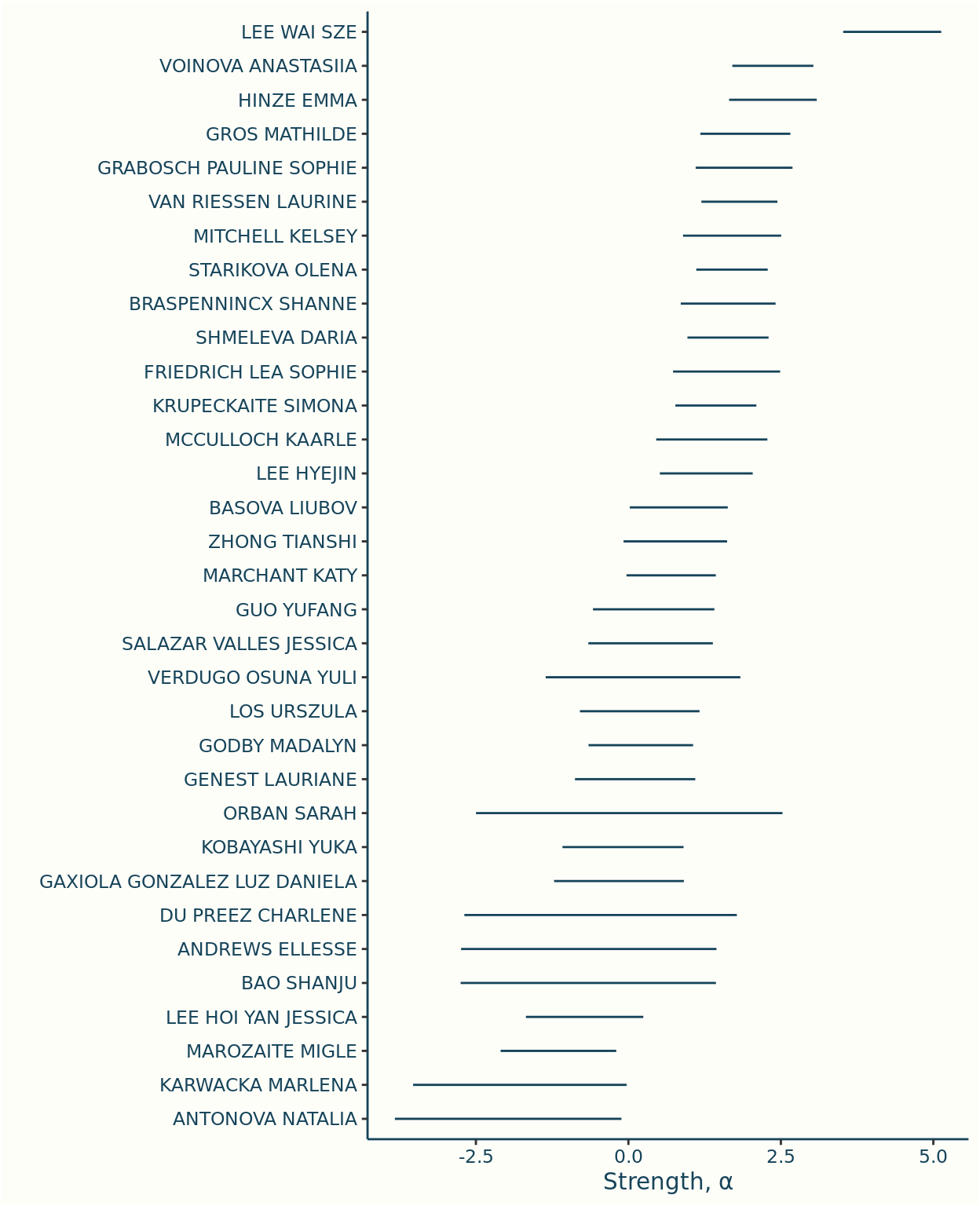There’s a compelling case for Lee Wai Sze to win gold - though this is based on the training data, so doesn’t include the latest results.

### Match Performance Metrics

In light of changing the likelihood, its worth reviewing the evaluation metrics I’m using.

I can either measure accuracy by the proportion of separate sprints that are correctly predicted, or the proportion of matches. I’ll stick with match outcomes, since when applying the model this will be the measure of interest.

The log-loss metric is intrinsically linked to the likelihood of the model (its the average log likelihood of the data), so we should revise this to use the new likelihood. This will also need a revised benchmark.

For a best-of-three match there are four possible outcomes (either athlete winning in either 2 or 3 sprints), so a random guessing strategy has a log loss of $$\log(\textstyle \frac14) \approx -1.39$$.

The benchmark for the full data is a weighted average of the single sprint benchmark and the best-of-three. Note that this means that the match log loss benchmark differs between the training and evaluation splits.

Accuracy
Data
Model Split 5% 50% (Median) 95%
bt1 evaluation 0.648 0.716 0.773
bt2 evaluation 0.648 0.716 0.773
bt3 evaluation 0.648 0.716 0.773
bt1 training 0.764 0.787 0.807
bt2 training 0.770 0.791 0.810
bt3 training 0.768 0.789 0.808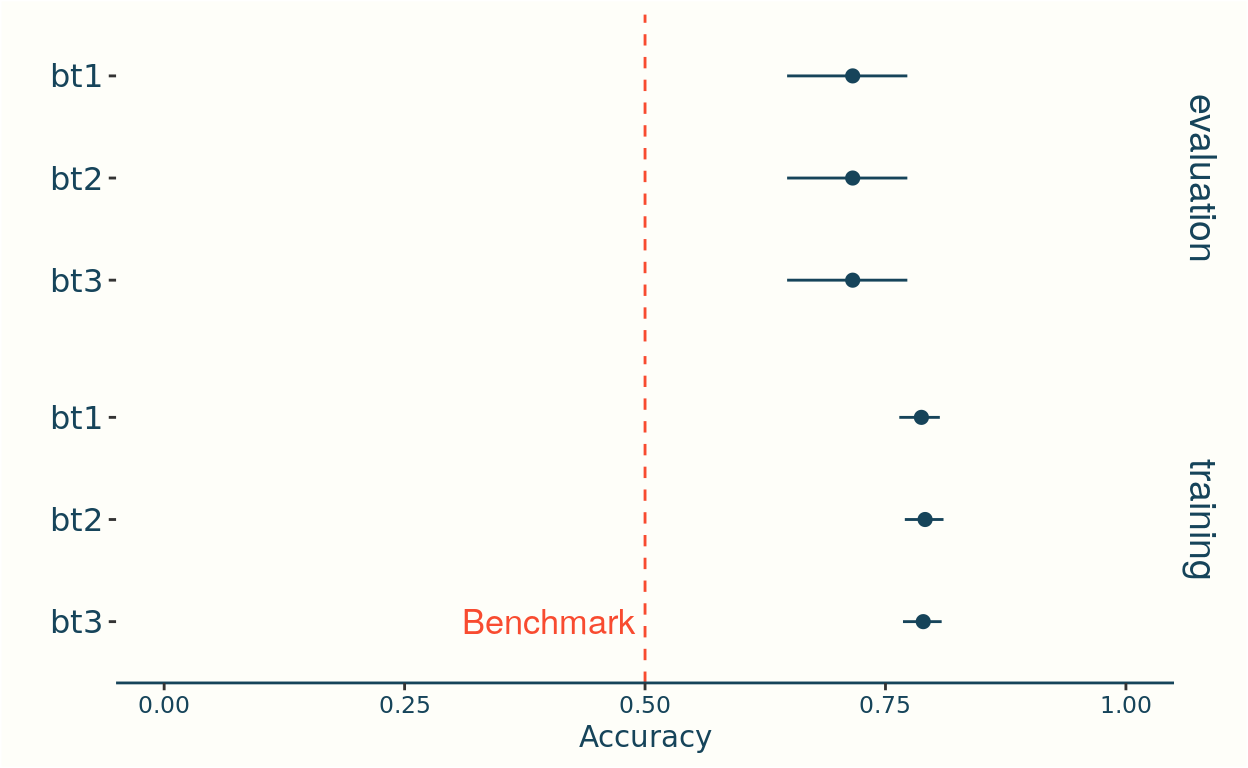Match Log Loss
Data
Model Split 5% 50% (Median) 95%
bt1 evaluation -1.087 -0.919 -0.800
bt2 evaluation -1.169 -0.951 -0.809
bt3 evaluation -1.096 -0.928 -0.809
bt1 training -0.642 -0.612 -0.586
bt2 training -0.635 -0.603 -0.575
bt3 training -0.612 -0.588 -0.567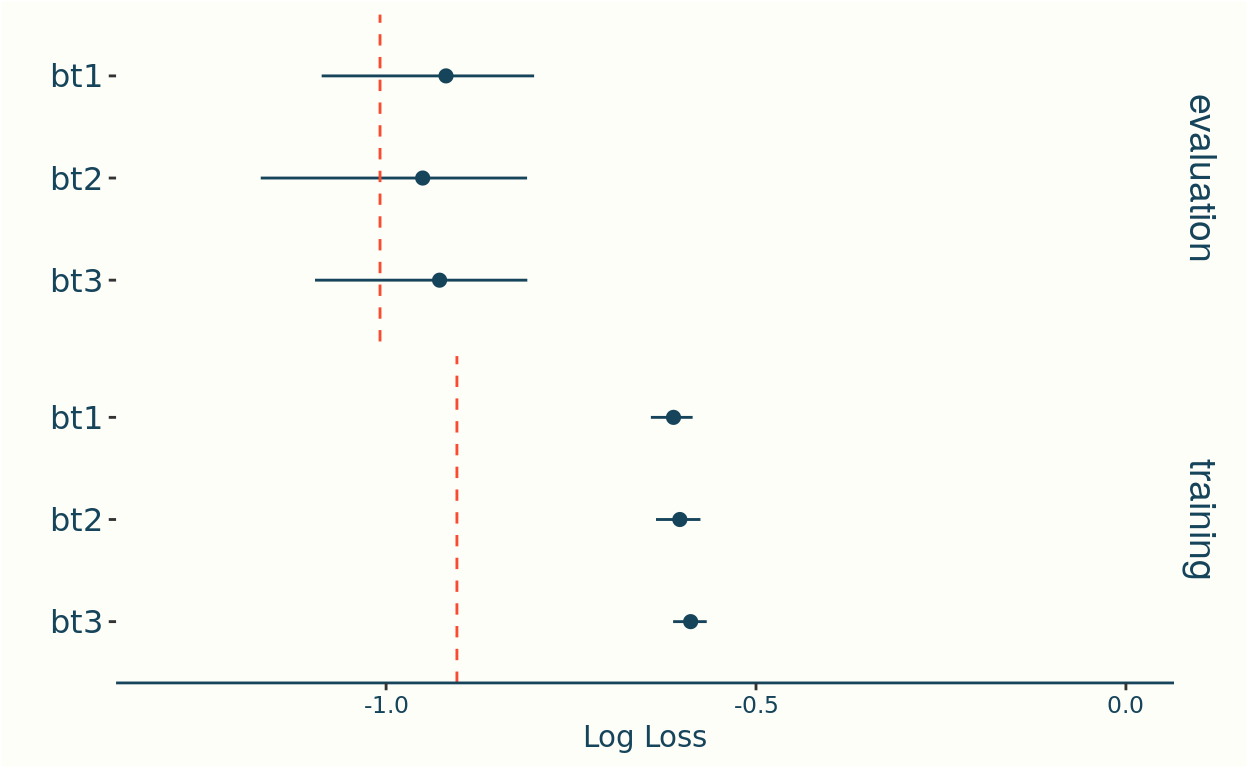Changing the likelihood has had a minimal impact on the evaluation metrics - with all three models defined to date performing similarly.

In hindsight this is to be expected as the volume of data that is evaluated differently under the revised likelihood is small: 86% of the training data are single sprints, for which both likelihoods agree, and only 2% have three sprints which is the scenario where the likelihoods differ the most.

I’ll carry this likelihood into the future models.

I didn’t have ready access to a list of each rider nationalities, but as a proxy I will use the nationality of the team they are riding for. I’ll consider a rider as being at home if the event is held in the country of their team.

The standard implementation of home advantage replaces the fixed strength $$\alpha_r$$, with a strength that varies by match:

$\alpha_{r}^{(m)} = \begin{cases} \alpha_r + \eta, &\text{ if r is at home in match m,} \\ \alpha_r , & \text{otherwise.} \end{cases}$

This assumes that all athletes feel the same benefitwhen competing at home, which I think is an over simplification. I’ll improve that assumption by using hierarchical modelling.

Hierarchical modelling allows us to vary the home advantage between riders, whilst accounting for the fact that we don’t have enough data to estimate each athlete’s effect independently.

The idea is to assume that there is some average effect, $$\eta$$, and that each rider then deviates from this average by some amount, $$\theta_r$$

$\eta_r = \eta + \theta_r.$

To help us define priors, I’ll consider the scenario where two riders with equal strength are competing, $$\alpha_w - \alpha_l = 0$$, and suppose one is at home. How much impact do we expect home advantage will have on the probability of winning?

No advantage would mean a win probability of 0.5, and I’d expect being at home might increase this to somewhere between 0.55 and 0.75. I can now turn that into a prior on $$\eta$$.

I also need a prior for the rider deviations $$\theta_r$$. Its reasonable to suppose this is normally distributed with mean 0, but we don’t know the appropriate standard deviation… and when in doubt, create a parameter!

I’ll let $$\upsilon$$ be this unknown standard deviation, so that $$\theta_r \sim \text{Normal}(0,\upsilon)$$, and put a prior on $$\upsilon$$.

Experimentation suggests priors $$\eta \sim \Gamma(2,4)$$ and $$\upsilon \sim \text{HalfNormal}(0,0.2)$$ align well to my heuristic above.

Parameter Priors

Show code
  home_effect_samples <- tibble(
eta = rgamma(1e05, shape = 2, rate = 4),
upsilon = abs(rnorm(1e05, mean = 0, sd =0.2))
) %>%
mutate(
p_win_avg = plogis(eta),
theta = rnorm(n(), mean = 0, sd = upsilon),
eta_theta = eta + theta,
p_win_rider = plogis(eta_theta)
)

home_param_prior <- home_effect_samples %>%
# convert to long format
select(eta, theta) %>%
gather(measure, sample, eta, theta) %>%
mutate(measure = if_else(measure == "eta", 'Average', 'Rider Deviation'))

ggplot(home_param_prior, aes(sample, y = ..density.., fill = measure)) +
geom_histogram(binwidth = 0.03, color = infreq_palette["beige"]) +
# scale_x_continuous(breaks = seq(0, 1, by=0.1)) +
coord_cartesian(xlim = c(-1,2)) +
labs(x = "Home Advantage", y = "") +
facet_grid(rows = vars(measure)) +
theme(
axis.line.y = element_blank(),
axis.text.y = element_blank(),
axis.ticks.y = element_blank(),
strip.background = element_blank(),  strip.text.y = element_text(size = 14),
legend.position = "none"
)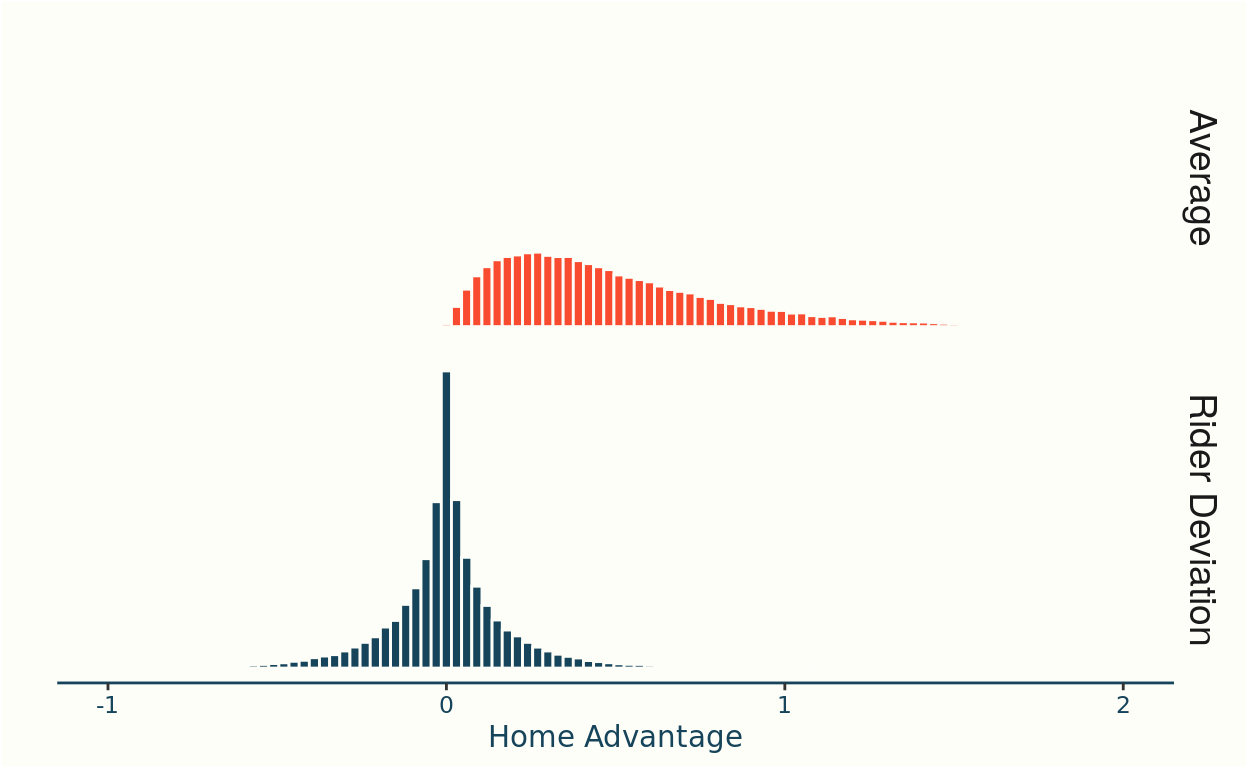Home Win Prior
Show code
home_wins_prior <- home_effect_samples %>%
# convert to long format
select(p_win_avg, p_win_rider) %>%
gather(measure, sample, p_win_avg, p_win_rider) %>%
mutate(measure = if_else(measure == "p_win_avg", 'Average', 'Rider'))

ggplot(home_wins_prior, aes(sample, y = ..density.., fill = measure)) +
geom_histogram(binwidth = 0.01, color = infreq_palette["beige"]) +
scale_x_continuous(breaks = seq(0, 1, by=0.1)) +
coord_cartesian(xlim = c(0,1)) +
labs(x = "Probability Home Rider Wins", y = "") +
facet_grid(rows = vars(measure)) +
theme(
axis.line.y = element_blank(),
axis.text.y = element_blank(),
axis.ticks.y = element_blank(),
strip.background = element_blank(),  strip.text.y = element_text(size = 14)
)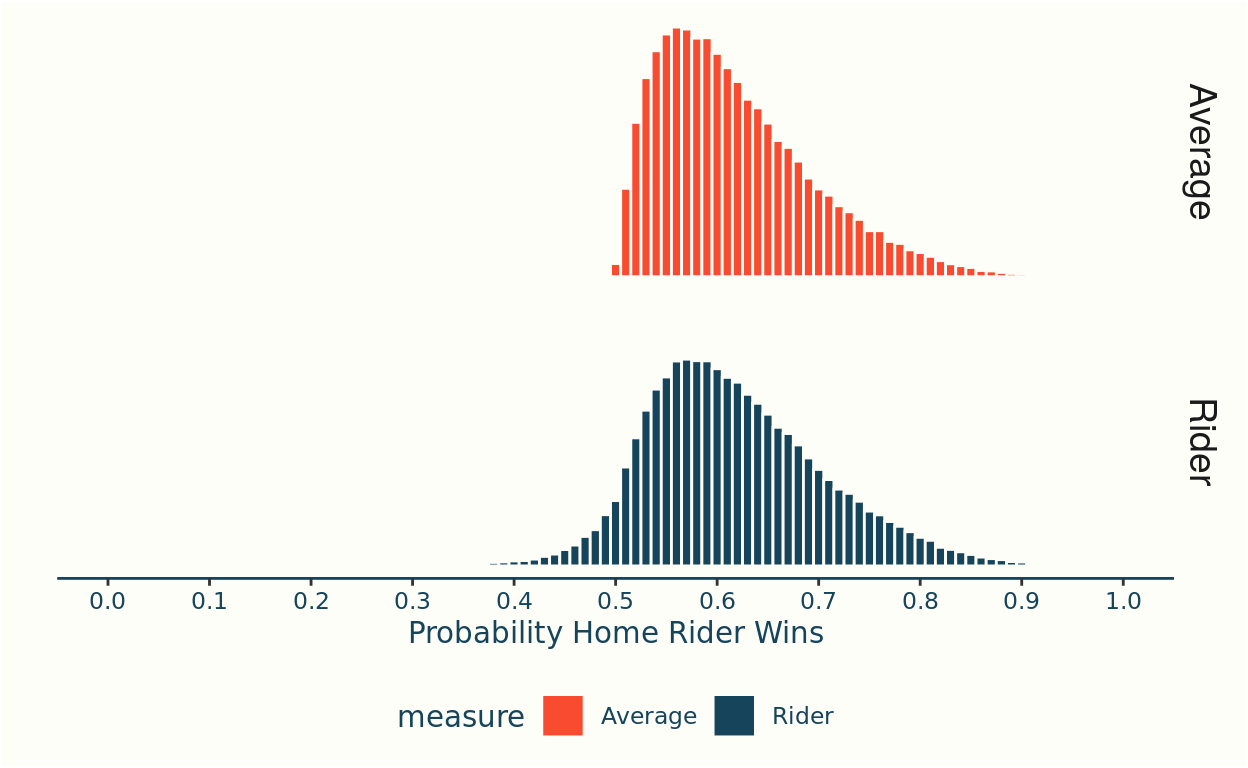BT4

I’ll follow the convention I introduced above, denoting $$\alpha_r^{(m)}$$ for a strength that varies by match. Further I’ll let $$\mathbf{1}_r^{(m)}$$ denote the indicator that equals 1 if the rider, r, is at home in the match, m.

\begin{align*} \bf{\text{Priors}} \\ \sigma & \sim \text{Gamma}(80,60) \\ \alpha_r & \sim \text{Normal}(0,\sigma) \\ \\ \eta & \sim \text{Gamma}(2,4) \\ \upsilon & \sim \text{Normal}(0, 0.2) \\ \theta_r & \sim \text{Normal}(0,\upsilon) \\ \\ \bf{\text{Likelihood}}\\ \alpha_r^{(m)} & = \alpha_r + (\eta + \theta_r)\mathbf{\large 1}_r^{(m)}\\ \alpha_w^{(m)} - \alpha_l^{(m)} | S & \sim L_{\text{match}}(\alpha_r^{(m)} - \alpha_s^{(m)} | S) \end{align*}

Example Data
Show code
# read match data from remote _target store

matches_sample <-  matches %>%
filter(event == '2020 UCI TRACK WORLD CYCLING CHAMPIONSHIPS', round == 'Finals', gender == 'Women') %>%
mutate(across(everything(), as.character)) %>%
select(event, date, gender, round, rider_1,rider_2,rider_id_1,rider_id_2, winner_id,loser_id, sprints, winner_at_home, loser_at_home) %>%
slice(1)

matches_sample <- tibble(Field = names(matches_sample), Example = unlist(matches_sample[1,]))
matches_sample %>%
kable("pipe") %>%
kable_styling(full_width=FALSE, bootstrap_options = c("striped", "hover", "condensed"),font_size = 10)

Field Example
event 2020 UCI TRACK WORLD CYCLING CHAMPIONSHIPS
date 2020-02-28
gender Women
round Finals
rider_1 HINZE EMMA
rider_2 VOINOVA ANASTASIIA
rider_id_1 44
rider_id_2 123
winner_id 44
loser_id 123
sprints 2
winner_at_home 1
loser_at_home 0

Stan code


functions {
real match_logit_lpmf(int[] s, vector delta){
real lpmf = 0;
vector[num_elements(delta)] p = inv_logit(delta);

for(n in 1:num_elements(s)){
if (s[n] == 1) lpmf += log(p[n]);
else if (s[n] == 2) lpmf += 2*log(p[n]);
else lpmf += log(2) + 2*log(p[n]) + log(1-p[n]);
}
return lpmf;
}

real accuracy(vector delta, int start, int end){
vector[end - start + 1] delta_seg = segment(delta, start, end - start + 1);
real acc = 0;

for(n in 1:num_elements(delta_seg)) acc += (delta_seg[n] > 0);

return inv(num_elements(delta_seg)) * acc;
}

real log_loss(vector delta, int start, int end){
vector[end - start + 1] delta_seg = segment(delta, start, end - start + 1);
real ll = 0;

return inv(num_elements(delta_seg)) * bernoulli_logit_lpmf(1 | delta_seg);
}

real match_log_loss(int[] s, vector delta, int start, int end){
int s_seg[end - start + 1]  = segment(s, start, end - start + 1);
vector[end - start + 1] delta_seg = segment(delta, start, end - start + 1);
real mll = 0;

return inv(num_elements(s_seg)) * match_logit_lpmf(s_seg | delta_seg);
}
}

data {
// Dimensions
int<lower=0> R; // Riders
int<lower=0> M; // Matches
int<lower=0,upper = M> T; // Training matches

// Match results
int<lower=1,upper=R> winner_id[M]; // ID's specifying riders in match
int<lower=1,upper=R> loser_id[M];
int<lower=1,upper=3> sprints[M];

real<lower=0,upper=1> winner_at_home[M];
real<lower=0,upper=1> loser_at_home[M];
}

parameters {
// rider ratings
real<lower=0> sigma;
vector[R] alpha0;

real<lower=0>eta;
real<lower=0>upsilon;
real theta[R];
}

transformed parameters {
// difference of winner and loser rating
vector[M] delta;
real eta_theta[R];

for(r in 1:R) eta_theta[r] = eta + theta[r];

for(m in 1:M){

delta[m] =
alpha0[winner_id[m]] - alpha0[loser_id[m]] +
(winner_at_home[m] * eta_theta[winner_id[m]]) - (loser_at_home[m] * eta_theta[loser_id[m]]);
}
}

model {
// (hierarchical) priors - strength
sigma ~ gamma(80,60);
alpha0 ~ normal(0,sigma);

// (hierarchical) priors - home effect
eta ~ gamma(2,4);
upsilon ~ normal(0,0.2);
theta ~ normal(0, upsilon);

// likelihood
}

generated quantities {
// maximum theoretical strength difference between two riders
real<lower=0> delta_max = max(alpha0) - min(alpha0);

// Calculate log-loss, match log-loss and accuracy. A separate value is returned
// for training/evaluation data, and within each of these the metrics are further
// broken down by round (5 rounds in total) and the total (6th entry in vectors).
real training_accuracy;
real evaluation_accuracy;
real training_match_log_loss;
real evaluation_match_log_loss;

training_accuracy = accuracy(delta,1, T);
training_match_log_loss = match_log_loss(sprints, delta, 1,T);
evaluation_accuracy = accuracy(delta, T+1, M);
evaluation_match_log_loss = match_log_loss(sprints, delta, T+1, M);
}

In Tokyo 2020 there’s a single female Japanese athlete, Yuka Kobayashi. The plot below shows the posterior ranges for the rider strengths, and shows the impact that the home advantage is expected to have (in blue).

Show code
bt4_draws <- read_remote_target("bt_draws_bt4")

japanese_riders <- olympic_riders %>% filter(Nationality == 'Japan') %>%
mutate(variable = paste0('theta[', rider_id,']'))

bt4_draws <- bt4_draws %>%
gather(variable, value, -starts_with("."))

bt4_rider_home_effect <- left_join(japanese_riders, bt4_draws) %>%
select(-variable) %>%
rename(rider_home_effect = value)

bt4_home_effect <- bt4_draws %>%
filter(str_detect(variable, '^eta')) %>%
rename(home_effect = value) %>%
select(-variable) %>%
left_join(bt4_rider_home_effect) %>%
mutate(rider_home_effect = rider_home_effect + home_effect)

bt4_strengths <- bt4_draws %>%
filter(str_detect(variable, 'alpha')) %>%
mutate(rider_id = str_match(variable, '\$(.*)\$')[,2] %>% as.numeric()) %>%
left_join(olympic_riders, .) %>%
left_join(bt4_home_effect) %>%
replace_na(list(rider_home_effect = 0))

bt4_strength_summ <- bt4_strengths %>%
group_by(rider_name) %>%
summarise(
is_not_home = all(is.na(home_effect )),
q5 = quantile(value, 0.05),
median = quantile(value, 0.5),
q95 = quantile(value, 0.95),
ha_q5 = quantile(value + rider_home_effect, 0.05),
ha_q95 = quantile(value + rider_home_effect, 0.95),
) %>%
mutate(rider_name = fct_reorder(rider_name,median))

ggplot(bt4_strength_summ) +
geom_segment(aes(x=q5,xend=q95,y=rider_name,yend=rider_name), color = infreq_palette["darkblue"]) +
geom_segment(data = bt4_strength_summ %>% filter(is_not_home == FALSE), aes(x=ha_q5,xend=ha_q95,y=rider_name,yend=rider_name), color = infreq_palette["orange"], size = 1.1) +
labs(y="",x="Strength, α")